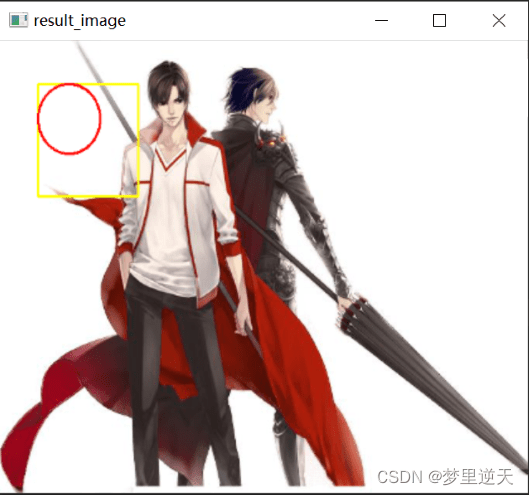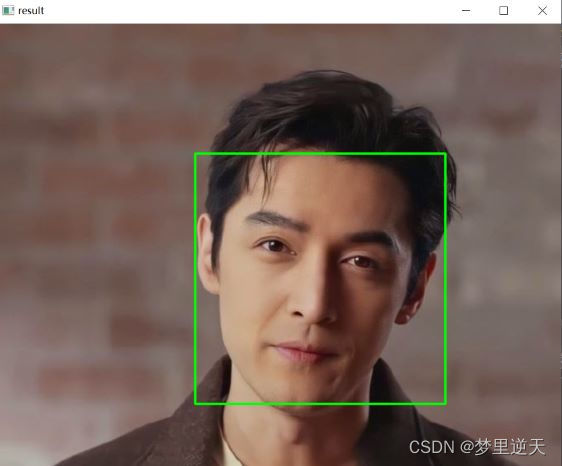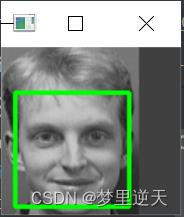# OpenCv人脸识别开发实战

## 一、OpenCV简介

OpenCV（全称：Open Source Computer Vision Library），是一个跨平台的计算机视觉库。OpenCV可用于开发实时的图像处理、计算机视觉以及模式识别程序。
​ OpenCV用C++语言编写，它的主要接口也是C++语言，但是依然保留了大量的C语言接口。该库也有大量的Python、Java和MATLAB/OCTAVE(2.5)的接口。如今也提供对于C#、Ch、Ruby、GO的支持。

## 二、OpenCV的基本使用

### 1.安装OpenCV模块

``````pip install opencv-python
``````

### 2.读取图片

``````# 导入模块
import cv2 as cv

# 读取图片
# 显示图片
# 等待键盘输入 单位毫秒 传入0则是无限等待
cv.waitKey(0)
# 由于OpenCV底层是C++编写的，需要释放内存
cv.destroyAllWindows()
``````### 3.图片灰度转换

OpenCV中有数百种关于在不同色彩空间之间转换的方法。当前，在计算机视觉中有三种常用的色彩空间：灰度、BGR以及HSV（Hue，Saturation，Value）。

• 灰度色彩空间是通过去除彩色信息来将其转换成灰阶，灰度色彩空间对中间处理特别有效，比如人脸识别。
• BGR即蓝、绿、红色彩空间，每一个像素点都由一个三元数组来表示，分别代表蓝、绿、红三种颜色。网页开发者可能熟悉另一个与之相似的颜色空间——RGB，它们只是颜色顺序不同。
• HSV，H（Hue）是色调，S（Saturation）是饱和度，V（Value）表示黑暗的程度（或光谱另一端的明亮程度）。
• 灰度转换的作用是：转换成灰度的图片的计算强度得以降低。
• 示例代码：

``````import cv2 as cv

# 加载图片
# 显示图片
cv.imshow('BGR image', img)
# cv2读取图片的通道是BGR（蓝绿红）
# PIL读取图片的通道是RGB
# 将图片灰度转换
gray_img = cv.cvtColor(img, code=cv.COLOR_BGR2GRAY)
# 显示转换后的图片
cv.imshow('gray_image', gray_img)
# 保存图片
cv.imwrite('gray_test.png', gray_img)
# 等待键盘输入
cv.waitKey(0)
# 释放内存
cv.destroyAllWindows()
``````

效果：### 4.修改图片尺寸

示例代码：

``````import cv2 as cv

# 加载图片

# 显示图片
# cv.imshow('input image', img)
print("原图片的形状", img.shape)
# 修改图片尺寸
# resize_img = cv.resize(img, dsize=(110, 160))
resize_img = cv.resize(img, dsize=(400, 360))
print("修改后图片的形状", resize_img.shape)
cv.imshow('resize_img', resize_img)
# 键盘输入q的时候，退出
while True:
if ord('q') == cv.waitKey(0):
break
# 释放内存
cv.destroyAllWindows()
``````

效果：### 5.绘制矩形、圆

OpenCV可以对图片进行任意编辑、处理。

示例代码

``````import cv2 as cv
# 左上角的坐标是(x,y)，矩形的宽度为w，高度为h
x, y, w, h = 50, 50, 80, 80
# 画矩形
cv.rectangle(img, (x, y, x+w, y+h), color=(0, 255, 255), thickness=2) # color=BGR，thickness参数表示画笔的粗细/线条宽度
# 画圆
cv.circle(img, center=(x+w//2, y+h//2), radius=w//2, color=(0, 0, 255), thickness=2)
resize_img = cv.resize(img, dsize=(420, 360))
# 显示图片
cv.imshow('result_image', resize_img)
cv.waitKey(0)
# 释放内存
cv.destroyAllWindows()
``````

效果：### 6.人脸检测

#### 6.1Haar级联的概念

由于灯光、视角、视距、摄像头抖动以及数字噪声的变化，图像细节变得不稳定。因此，提取出图像的细节对产生稳定分类结果和跟踪结果很有用。这些提取的结果被称为特征，专业的表述为：从图像数据中提取特征。两个图像的相似程度可以通过它们对应特征的欧氏距离来度量。
Haar特征是一种用于实现人脸跟踪的特征。每一个Haar特征都描述了相邻图像区域的对比模式。例如，边、顶点和细线都能生成具有判别性的特征。

#### 6.2获取Haar级联数据

OpenCV提供了人脸检测所需的XML文件，可用于检测静止图像、视频和摄像头所得到图像中的人脸。

• #### 6.3使用OpenCV进行人脸检测

##### 6.3.1静态图像中人脸检测
###### 6.3.1.1检测人脸

示例代码：

``````import cv2 as cv

def face_detect_demo():
# 将图片转换为灰度
gray = cv.cvtColor(img, cv.COLOR_BGR2GRAY)
# 加载特征数据
# faces为检测区域
faces = face_detector.detectMultiScale(gray)
for x, y, w, h in faces:
# 绘制矩形
cv.rectangle(img, (x, y), (x+w, y+h), color=(0, 255, 0), thickness=2)
# 显示图片
cv.imshow('result', img)

# 加载图片
face_detect_demo()
# cv.imshow('input image', img)
cv.waitKey(0)
cv.destroyAllWindows()
``````

效果：###### 6.3.1.2检测多张人脸

示例代码：

``````import cv2 as cv

# 定义人脸检测方法
def face_detect_demo():
# 将图片灰度
gray = cv.cvtColor(img, cv.COLOR_BGR2GRAY)
# 加载特征数据
# faces为检测区域
# 发现有一些没检测出来
faces = face_detector.detectMultiScale(gray, scaleFactor=1.01, minNeighbors=3, maxSize=(40, 40), minSize=(35, 35))
for x, y, w, h in faces:
print(x, y, w, h)
cv.rectangle(img, (x, y), (x+w, y+h), color=(0, 0, 255), thickness=2)
cv.circle(img, center=(x+w//2, y+h//2), radius=w//2, color=(0, 255, 0), thickness=2)
# 显示图片
cv.imshow('result', img)

# 加载图片
# 调用人脸检测方法
face_detect_demo()
cv.waitKey(0)
cv.destroyAllWindows()
``````

测试图片：效果：##### 6.3.2视频中的人脸检测

视频是一张张图片组成的，在视频的帧上重复这个过程就能完成视频中的人脸检测。

示例代码：

``````import cv2 as cv

def face_detect_demo(img):
# 将图片转换为灰度
gray = cv.cvtColor(img, cv.COLOR_BGR2GRAY)
# 加载特征数据
# faces为检测区域
for x, y, w, h in faces:
# 绘制矩形
cv.rectangle(img, (x, y), (x + w, y + h), color=(0, 0, 255), thickness=2)
cv.circle(img, center=(x+w//2, y+h//2), radius=w//2, color=(0, 255, 0), thickness=2)

# 可调整窗口大小
cv.namedWindow('result', 0)
# 调整窗口大小
cv.resizeWindow('result', 500, 500)
# 显示图片
cv.imshow('result', img)

# 读取视频
cap = cv.VideoCapture('test.mp4')
while True:
# frame就是每一帧的图像，是个三维矩阵（按帧读取）
print(f'flag:{flag}, frame.shape:{frame.shape}')
if not flag:
# 视频播放结束，退出
break
face_detect_demo(frame)
if ord('q') == cv.waitKey(0):
break
# 释放内存
cv.destroyAllWindows()
cap.release()
``````

效果：##### 6.3.3人脸识别

人脸识别其实就是一个程序能识别给定图像或视频中的人脸。实现这一目标的方法之一是用一系列分好类的图像来”训练“程序，并基于这些图像来进行识别。这就是OpenCV及其人脸识别模块进行人脸识别的过程。
人脸识别模块的另一个重要特征是：每个识别都具有转置信评分，因此可在实际应用中通过对其设置阈值来进行筛选。
人脸识别所需要的人脸可以通过两种方式来得到：自己获得图像或从人脸数据库免费获得可用的人脸图像。

###### 6.3.3.1训练数据

有了数据，需要将这些样本图像加载到人脸识别算法中。所有的人脸识别算法在它们的train()函数都有两个参数：图像数组和标签数组。这些标签表示进行识别时某人人脸的ID，因此根据ID可以知道被识别的人是谁。要做到这一点，将在”trainer/“目录中保存为.yml文件。

在使用Python 3&OpenCV 3.0.0进行人脸识别训练时发现异常：
`AttributeError:'module' object has no attribute 'LBPHFaceRecognizer_create' OpenCV` ，需要安装opencv-contrib-python模块：

``````pip install opencv-contrib-python
``````

示例代码：

``````import os
import cv2

import numpy as np
from PIL import Image

def getImageAndLabels(path):
facesSamples = []
ids = []
imagePaths = [os.path.join(path, f) for f in os.listdir(path)]
# 检测人脸
# 遍历列表中的图片
for imagePath in imagePaths:
# 打开图片
PIL_img = Image.open(imagePath).convert('L')
# 将图像转换为数组
img_numpy = np.array(PIL_img, 'uint8')
# faces为检测区域
faces = face_detector.detectMultiScale(img_numpy)
# 获取每张图片的id
id = int(os.path.split(imagePath).split('.'))
for x, y, w, h in faces:
facesSamples.append(img_numpy[y:y+h, x:x+w])
ids.append(id)
return facesSamples, ids

if __name__ == '__main__':
# 图片路径
path = './data/jm/'
# 获取图像数组和id标签数组
faces, ids = getImageAndLabels(path)
# 获取循环对象
"""
AttributeError: module 'cv2' has no attribute 'face'
"""
recognizer = cv2.face.LBPHFaceRecognizer_create()
recognizer.train(faces, np.array(ids))
# 保存文件
recognizer.write('trainer/trainer.yml')
``````

训练部分图像：###### 6.3.3.2基于LBPH的人脸识别

LBPH(Local Binary Pattern Histogram)将检测到的人脸分为小单元，并将其与模型中的对应单位进行比较，对每个区域的匹配值产生一个直方图。由于这种方法的灵活性，LBPH是唯一允许模型样本人脸和检测到的人脸在形状、大小上可以不同的人脸识别算法。
调整后的区域中调用predict()函数，该函数返回两个元素的数组：第一个元素是所识别个体的标签，第二个是置信度评分。所有的算法都有一个置信度评分阈值，置信度评分用来衡量所识别人脸与原模型的差距，0表示完全匹配。
有时不想保留所有的识别结果，则需要进一步处理，因此可用自己的算法来估算识别的置信度评分。LBPH一个好的识别参考值要低于50，任何一个高于80的参考值都会被认为是低的置信度评分。

示例代码：

``````import cv2

# 加载训练数据集文件
recognizer = cv2.face.LBPHFaceRecognizer_create()

# 准备识别的图片
# 将图片转换为灰度
gray = cv2.cvtColor(img, cv2.COLOR_BGR2GRAY)
# 加载特征数据
# faces为检测区域
faces = face_detector.detectMultiScale(gray)
for x, y, w, h in faces:
# 绘制矩形
cv2.rectangle(img, (x, y), (x + w, y + h), color=(0, 255, 0), thickness=2)
# 人脸识别
id,confidence = recognizer.predict(gray[y:y+h, x:x+w])
print(f"标签id：{id}，置信评分：{confidence}")
# 显示图片
cv2.imshow('result', img)
cv2.waitKey(0)
# 释放内存
cv2.destroyAllWindows()
``````

测试图像：测试效果：因为上面这个图像不在训练数据中，所以置信评分高于80。

换成下面这个图像（jim文件夹下的11.bmp）进行测试：测试效果：在训练数据中，置信评分为0。#### 参考文章及其它：

• https://blog.csdn.net/weixin_43493559/article/details/106600471
• B站视频：OpenCv人脸识别开发实战
• https://blog.csdn.net/shangxiaqiusuo1/article/details/84888408
• ORL人脸数据库40个：http://www.qudong51.net/qudong/1040.html
• http://www.webzuan.cn/szk/7194.html
• https://blog.csdn.net/qq_52131774/article/details/121265931
• 来源：梦里逆天

物联沃分享整理
物联沃-IOTWORD物联网 » OpenCv人脸识别开发实战